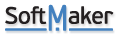## IF function help needed

snowshed
Posts: 56
Joined: Fri Feb 23, 2018 11:10 pm

### IF function help needed

It's been 50+ years since I needed to write any complex algebraic formulas, so the memory is not so great. LOL

I'm creating a spreadsheet listing all the various screen resolutions I can find. Once the resolutions are known, I want to calculate the aspect ratio of those resolutions.

For example, I put 1920 in a cell, and 1080 in another cell. Then in a 3rd cell, the aspect ratio of 16:9 is displayed.

The following formula, when placed in the 3rd cell, will give me a result of 16:9 when 1920 and 1080 are inserted in the first and second cells.

=\$A27/GCD(\$A27,\$B27)&":"&\$B27/GCD(\$A27,\$B27)

Every other resolution I've found and tried results in the correct aspect ratio.

Except... 1920 X 1200, and others. That gives you a result of 8:5, which, while technically correct, those are not the "numbers" you see listed in monitor specs. You see 16:10 in the specs.

When I enter these numbers, or similar, I'd like the result in the third cell to be 16:10, not 8:5.

It occurred to me the IF (if-th-else) function could be used to display 16:10 when the computations come out to 8:5. And if the math doesn't give you 8:5, it will display the correct aspect ratio.

Can it be done? How do I create the formula?

Thanks.

miguel-c
SoftMaker TeamPosts: 597
Joined: Wed Jun 05, 2019 12:02 pm

### Re: IF function help needed

You can nest IFs and the solution to your problem is:
=IF((\$A27/GCD(\$A27,\$B27)&":"&\$B27/GCD(\$A27,\$B27))="8:5","16:10",(\$A27/GCD(\$A27,\$B27)&":"&\$B27/GCD(\$A27,\$B27)))

snowshed
Posts: 56
Joined: Fri Feb 23, 2018 11:10 pm

### Re: IF function help needed

miguel-c wrote:
Fri Aug 02, 2019 4:50 pm
You can nest IFs and the solution to your problem is:
=IF((\$A27/GCD(\$A27,\$B27)&":"&\$B27/GCD(\$A27,\$B27))="8:5","16:10",(\$A27/GCD(\$A27,\$B27)&":"&\$B27/GCD(\$A27,\$B27)))
Thanks, Miiguel, exactly what I was looking for.

And, I was so close in my experimenting. All I was missing are the quotation marks around 8:5 and 16:10.